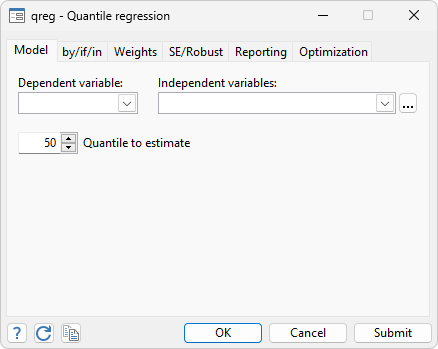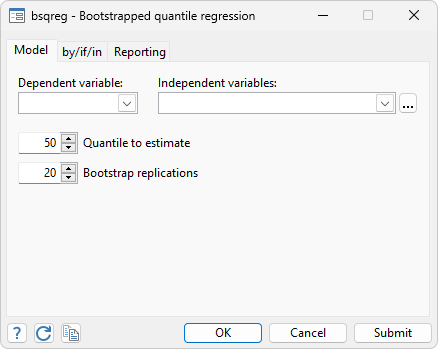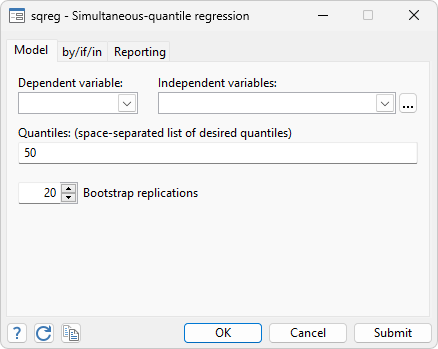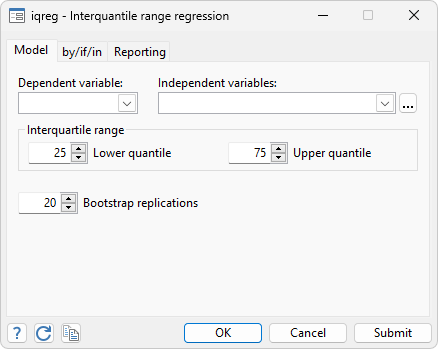Home  /  Products  /  Features  /  Linear models  /  Quantile regression

## Quantile regression

• Including median, minimization of sums of absolute deviations
• There are now three ways to obtain the VCE:
• the standard Koenker and Bassett method appropriate for i.i.d. errors;
• a Huber sandwich estimator that can be used even if the errors are not i.i.d.;
• the bootstrap.
For the first two VCE methods above, there are many choices of bandwidth methods and kernels to select from.Stata fits quantile (including median) regression models, also known as least-absolute value (LAV) models, minimum absolute deviation (MAD) models, and L1-norm models.

Median regression estimates the median of the dependent variable, conditional on the values of the independent variable. This is similar to least-squares regression, which estimates the mean of the dependent variable. Said differently, median regression finds the regression plane that minimizes the sum of the absolute residuals rather than the sum of the squared residuals.

. webuse auto
(1978 automobile data)

. qreg price weight length foreign
Iteration 1:  WLS sum of weighted deviations =  56397.829

Iteration 1:  Sum of abs. weighted deviations =    55950.5
Iteration 2:  Sum of abs. weighted deviations =  55264.718
Iteration 3:  Sum of abs. weighted deviations =  54762.283
Iteration 4:  Sum of abs. weighted deviations =  54734.152
Iteration 5:  Sum of abs. weighted deviations =  54552.638
note: alternate solutions exist.
Iteration 6:  Sum of abs. weighted deviations =  54465.511
Iteration 7:  Sum of abs. weighted deviations =  54443.699
Iteration 8:  Sum of abs. weighted deviations =  54411.294

Median regression                                   Number of obs =         74
Raw sum of deviations  71102.5 (about 4934)
Min sum of deviations 54411.29                    Pseudo R2     =     0.2347

price   Coefficient  Std. err.      t    P>|t|     [95% conf. interval]

weight     3.933588   1.328718     2.96   0.004     1.283543    6.583632
length    -41.25191   45.46469    -0.91   0.367    -131.9284    49.42456
foreign     3377.771   885.4198     3.81   0.000     1611.857    5143.685
_cons     344.6489   5182.394     0.07   0.947     -9991.31    10680.61



By default, qreg performs median regression—the estimates above were obtained by minimizing the sums of the absolute residuals.

By comparison, the results from least-squares regression are

. regress price weight length foreign

Source         SS           df       MS    Number of obs   =        74
F(3, 70)        =     28.39
Model     348565467         3   116188489   Prob > F        =    0.0000
Residual     286499930        70  4092856.14   R-squared       =    0.5489
Total     635065396        73  8699525.97   Root MSE        =    2023.1

price   Coefficient  Std. err.      t    P>|t|     [95% conf. interval]

weight     5.774712   .9594168     6.02   0.000     3.861215    7.688208
length    -91.37083   32.82833    -2.78   0.007    -156.8449   -25.89679
foreign     3573.092    639.328     5.59   0.000     2297.992    4848.191
_cons     4838.021    3742.01     1.29   0.200    -2625.183    12301.22



qreg can also estimate the regression plane for quantiles other than the 0.5 (median). For instance, the following model describes the 25th percentile (.25 quantile) of price:

. qreg price weight length foreign, quantile(.25)
Iteration 1:  WLS sum of weighted deviations =  49469.235

Iteration 1:  Sum of abs. weighted deviations =  49728.883
Iteration 2:  Sum of abs. weighted deviations =   45669.89
Iteration 3:  Sum of abs. weighted deviations =  43416.646
Iteration 4:  Sum of abs. weighted deviations =  41947.221
Iteration 5:  Sum of abs. weighted deviations =  41093.025
Iteration 6:  Sum of abs. weighted deviations =  37623.424
Iteration 7:  Sum of abs. weighted deviations =  35721.453
Iteration 8:  Sum of abs. weighted deviations =  35226.308
Iteration 9:  Sum of abs. weighted deviations =  34823.319
Iteration 10: Sum of abs. weighted deviations =  34801.777

.25 Quantile regression                             Number of obs =         74
Raw sum of deviations 41912.75 (about 4187)
Min sum of deviations 34801.78                    Pseudo R2     =     0.1697

price   Coefficient  Std. err.      t    P>|t|     [95% conf. interval]

weight      1.831789   .6328903     2.89   0.005     .5695289    3.094049
length       2.84556   21.65558     0.13   0.896    -40.34514    46.03626
foreign      2209.925   421.7401     5.24   0.000     1368.791    3051.059
_cons     -1879.775    2468.46    -0.76   0.449    -6802.963    3043.413



Here, we perform median regression but request robust standard errors.

. qreg price weight length foreign, vce(robust)
Iteration 1:  WLS sum of weighted deviations =  56397.829

Iteration 1:  Sum of abs. weighted deviations =    55950.5
Iteration 2:  Sum of abs. weighted deviations =  55264.718
Iteration 3:  Sum of abs. weighted deviations =  54762.283
Iteration 4:  Sum of abs. weighted deviations =  54734.152
Iteration 5:  Sum of abs. weighted deviations =  54552.638
note: alternate solutions exist.
Iteration 6:  Sum of abs. weighted deviations =  54465.511
Iteration 7:  Sum of abs. weighted deviations =  54443.699
Iteration 8:  Sum of abs. weighted deviations =  54411.294

Median regression                                   Number of obs =         74
Raw sum of deviations  71102.5 (about 4934)
Min sum of deviations 54411.29                    Pseudo R2     =     0.2347

Robust
price   Coefficient  std. err.      t    P>|t|     [95% conf. interval]

weight     3.933588   1.694477     2.32   0.023       .55406    7.313116
length    -41.25191   51.73571    -0.80   0.428    -144.4355    61.93171
foreign     3377.771   728.5115     4.64   0.000     1924.801    4830.741
_cons     344.6489   5096.528     0.07   0.946    -9820.055    10509.35



Stata can provide bootstrapped standard errors, using the bsqreg command. set seed 1001

. bsqreg price weight length foreign
(fitting base model)

Bootstrap replications (20)

1   2   3   4   5

....................

Median regression, bootstrap(20) SEs                Number of obs =         74
Raw sum of deviations  71102.5 (about 4934)
Min sum of deviations 54411.29                    Pseudo R2     =     0.2347

price   Coefficient  Std. err.      t    P>|t|     [95% conf. interval]

weight     3.933588   2.941839     1.34   0.186    -1.933726    9.800901
length    -41.25191   73.47105    -0.56   0.576    -187.7853    105.2815
foreign     3377.771   1352.518     2.50   0.015     680.2582    6075.284
_cons     344.6489   5927.045     0.06   0.954    -11476.47    12165.77



The coefficient estimates are the same as those in the first example. The standard errors, and, therefore, the t statistics, significance levels, and confidence intervals differ.

Stata can also perform simultaneous-quantile regression. With simultaneous-quantile regression, we can estimate multiple quantile regressions simultaneously:. set seed 1001

. sqreg price weight length foreign, q(.25 .5 .75)
(fitting base model)

Bootstrap replications (20)

1   2   3   4   5

....................

Simultaneous quantile regression                    Number of obs =         74
bootstrap(20) SEs                                 .25 Pseudo R2 =     0.1697
.50 Pseudo R2 =     0.2347
.75 Pseudo R2 =     0.3840

Bootstrap
price    Coefficient  std. err.      t    P>|t|     [95% conf. interval]

q25
weight       1.831789   1.250388     1.46   0.147    -.6620304    4.325608
length        2.84556   24.53036     0.12   0.908     -46.0787    51.76982
foreign       2209.925   1099.174     2.01   0.048      17.6916    4402.159
_cons      -1879.775   3087.115    -0.61   0.545    -8036.831    4277.282

q50
weight       3.933588   2.153228     1.83   0.072    -.3608896    8.228065
length      -41.25191   55.61779    -0.74   0.461    -152.1781    69.67427
foreign       3377.771    1151.72     2.93   0.005     1080.738    5674.804
_cons       344.6489   5152.738     0.07   0.947    -9932.164    10621.46

q75
weight        9.22291   2.315138     3.98   0.000     4.605513    13.84031
length      -220.7833   83.26476    -2.65   0.010    -386.8496   -54.71695
foreign       3595.133   1072.378     3.35   0.001     1456.342    5733.924
_cons        20242.9   9612.649     2.11   0.039     1071.081    39414.73



We can test whether the effect of weight is the same at the 25th and 75th percentiles:

. test[q25]weight = [q75]weight

( 1)  [q25]weight - [q75]weight = 0

F(  1,    70) =   12.59
Prob > F =    0.0007


We can obtain a confidence interval for the difference in the effect of weight at the 25th and 75th percentiles:

. lincom [q75]weight-[q25]weight

( 1)  - [q25]weight + [q75]weight = 0

price    Coefficient  Std. err.      t    P>|t|     [95% conf. interval]

(1)      7.391121   2.082689     3.55   0.001     3.237329    11.54491



Stata also performs interquantile regression, which focuses on one quantile comparison:. set seed 1001

. iqreg price weight length foreign, q(.25 .75)
(fitting base model)

Bootstrap replications (20)

1   2   3   4   5

....................

.75-.25 Interquantile regression                    Number of obs =         74
bootstrap(20) SEs                                 .75 Pseudo R2 =     0.3840

Bootstrap
price    Coefficient  std. err.      t    P>|t|     [95% conf. interval]

weight      7.391121   2.082689     3.55   0.001     3.237329    11.54491
length     -223.6288   74.62895    -3.00   0.004    -372.4716   -74.78609
foreign      1385.208   1420.119     0.98   0.333     -1447.13    4217.545
_cons      22122.68   9288.568     2.38   0.020     3597.215    40648.14



### References

Gould, W. 1992.
sg11.1: Quantile regression with bootstrapped standard errors. Stata Technical Bulletin 9: 19–21. Reprinted in Stata Technical Bulletin Reprints, vol. 2, pp. 137–150.
Gould, W., and W. H. Rogers. 1994.
Quantile regression as an alternative to robust regression. Proceedings of the Statistical Computing Section. Alexandria, VA: American Statistical Association.
Hao, Lingxin, and Daniel Q. Naiman. 2007.
Quantile regression.
Rogers, W. H. 1992.
sg11: Quantile regression standard errors. Stata Technical Bulletin 9: 16–19. Reprinted in Stata Technical Bulletin Reprints, vol. 2, pp. 133–137.
------. 1993.
sg11.2: Calculation of quantile regression standard errors. Stata Technical Bulletin 13: 18–19. Reprinted in Stata Technical Bulletin, vol. 3, pp. 77–78.